##### Geometry Workbook For Dummies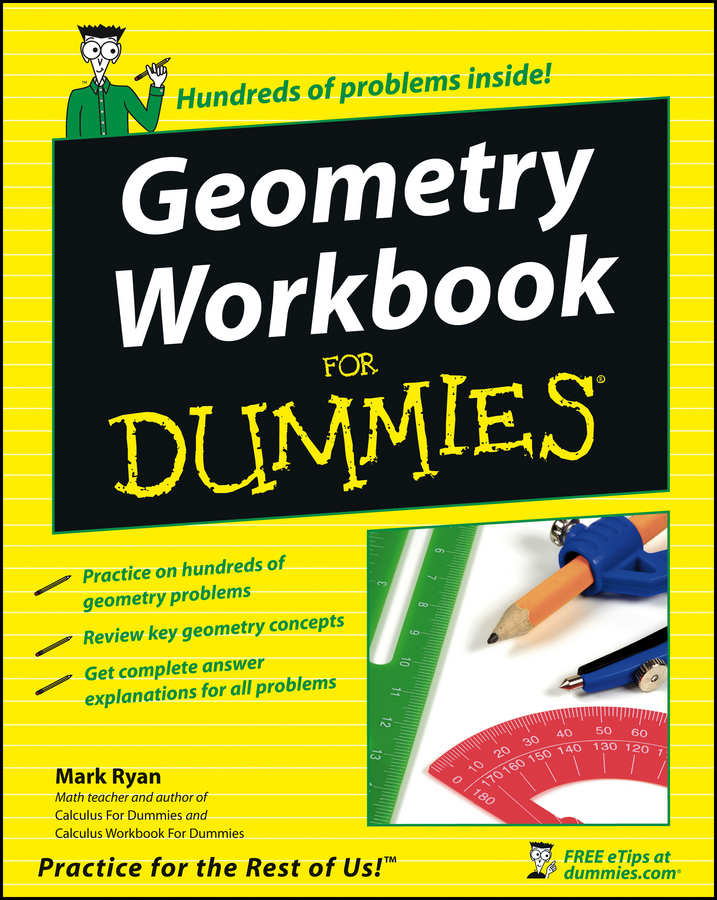You can solve certain similar triangle problems using the Side-Splitter Theorem. This theorem states that if a line is parallel to a side of a triangle and it intersects the other two sides, it divides those sides proportionally. See the below figure.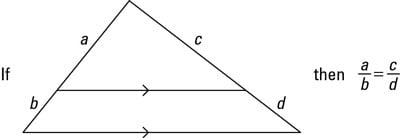Check out the following problem, which shows this theorem in action: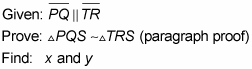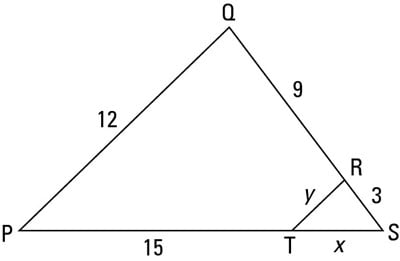Here’s the proof: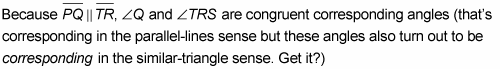Then, because both triangles contain angle S, the triangles are similar by AA (Angle-Angle).

Now find x and y.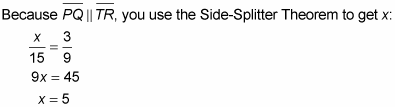And here’s the solution for y: First, don’t fall for the trap and conclude that y = 4. Side y looks like it should equal 4 for two reasons: First, you could jump to the erroneous conclusion that triangle TRS is a 3-4-5 right triangle. But nothing tells you that triangle TRS is a right angle, so you can’t conclude that.

Second, when you see the ratios of 9 : 3 (along segment QS) and 15 : 5 (along segment PS, after solving for x), both of which reduce to 3 : 1, it looks like PQ and y should be in the same 3 : 1 ratio. That would make PQ : y a 12 : 4 ratio, which again leads to the wrong answer that y is 4. The answer comes out wrong because this thought process amounts to using the Side-Splitter Theorem for the sides that aren’t split — which you aren’t allowed to do.

Don’t use the Side-Splitter Theorem on sides that aren’t split. You can use the Side-Splitter Theorem only for the four segments on the split sides of the triangle. Do not use it for the parallel sides, which are in a different ratio. For the parallel sides, use similar-triangle proportions. (Whenever a triangle is divided by a line parallel to one of its sides, the triangle created is similar to the original, large triangle.)

So finally, the correct way to get y is to use an ordinary similar-triangle proportion. The triangles in this problem are positioned the same way, so you can write the following: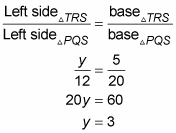That’s a wrap.